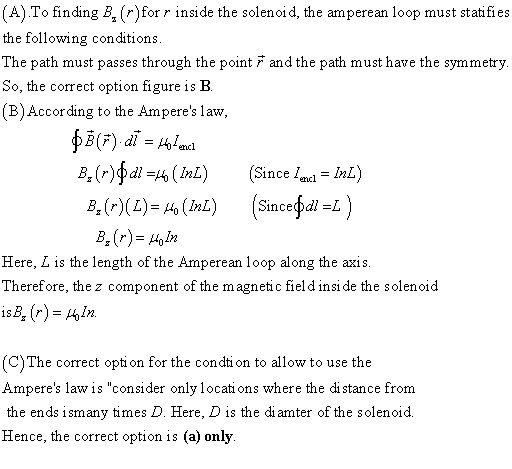Question# A.Which figure shows the loop that the must beused as the Ampèrean loop for finding for inside the solenoid? B.Find , the z component of the magnetic

Other
ANSWEREDA.Which figure shows the loop that the must beused as the Ampèrean loop for finding for inside the solenoid?
B.Find , the z component of the magnetic field insidethe solenoid where Ampère's law applies. Express your answer in terms of,,,,and physical constants such as .
C.The magnetic field inside a solenoidcan be found exactly using Ampère's law only if thesolenoid is infinitely long. Otherwise, the Biot-Savart law must beused to find an exact answer. In practice, the field can bedetermined with very little error by using Ampère's law, aslong as certain conditions hold that make the field similar to thatin an infinitely long solenoid. Which of the following conditions musthold to allow you to use Ampère's law to find a goodapproximation?
Consider only locations where the distance from the ends ismany times . Consider any location inside the solenoid, as long asis much larger than for the solenoid. Consider only locations along the axis of the solenoid. Answer?
Show transcribed image text A. Which figure shows the loop that the must beused as the Amp?rean loop for finding B_z(r) for r inside the solenoid? B. Find B_z(r), the z component of the magnetic field insidethe solenoid where Amp?re's law applies. Express your answer in terms ofL,D,n,I,and physical constants such as mu_0. C. The magnetic field inside a solenoidcan be found exactly using Amp?re's law only if thesolenoid is infinitely long. Otherwise, the Biot - Savart law must beused to find an exact answer. In practice, the field can bedetermined with very little error by using Amp?re's law, aslong as certain conditions hold that make the field similar to thatin an infinitely long solenoid. Which of the following conditions musthold to allow you to use Amp?re's law to find a goodapproximation? Consider only locations where the distance from the ends ismany times D. Consider any location inside the solenoid, as long asLis much larger than Dfor the solenoid. Consider only locations along the axis of the solenoid.